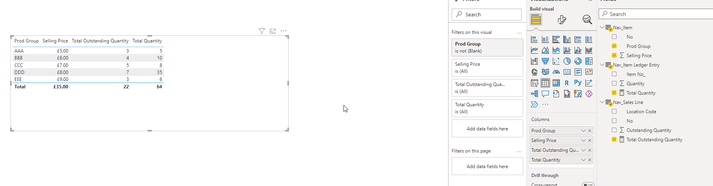cancel
Showing results for
Did you mean:Helper III

## DAX measure total not computing my changed value (Has been a pain for me I can't get)

Hi Everyone,

Very simplified but could someone explain why this isn't being computed properly? This has been frying my brain for days.😵

I need the solution as a measure can't be a calculated column or done in Power Query I need the solution as a measure.

Here are my tables and my measures below.....

Table1

 ID Bingo A -5 B -20 C -90 D 5 E 10 F 15 G 0

Total Bingo = SUM('Table'[Bingo])

No Negatives = IF('Table'[Total Bingo] < 0,0,'Table'[Total Bingo])

End result...I need all negative values turned into 0's and then the total at the bottom. I would love to do this in power query but my actual reports problem boils down to this same issue and the end result has been built upon measures so the solution in a measure would be extremly helpful.

Thanks in advance all you would be a life saver!

Many Thanks,

Taylor

1 ACCEPTED SOLUTIONSuper User

You can use

``````No negatives =
SUMX (
'Nav_item',
VAR Quantity =
CALCULATE ( SUM ( 'Nav_item ledger entry'[Quantity] ) )
VAR OutstandingQuantity =
CALCULATE ( SUM ( 'Nav_sales line'[Outstanding quantity] ) )
VAR Difference =
MAX ( OutstandingQuantity - Quantity, 0 )
RETURN
Difference * 'Nav_item'[Selling price]
)
``````
10 REPLIES 10Helper III

Would you guys be able to build it with these?

Table: Nav_Sales

ID: No

Table: Nav_Item

ID: No

Table Name: Nav_Item Ledger

ID Name: No

Relationships-

Nav_Item Legder -> Nav_Item = Many to One

Nav_Sales -> Nav_Item = Many to One

This is such great help thanks guys.

Many Thanks,

TaylorSuper User

which columns from which tables are involved, and what is the formula ? is it the sum of a column from one table multiplied by the sum of a column from the other table, for the same nav item ?Helper III

Hi @johnt75 ,

This is it here...Relationship has be be like this because this is just a sample from the tables of columns that I've needed.

I need to do: Total Outstanding Quantity -  Total Quantity

And then assign all negative values from this equation to zeros, then multiply this equation by the Selling price.

Here are my Tables...

 Item No Quantity 1 5 2 10 3 8 4 35 5 6 6 15 7 16 8 17 9 18 10 19

 No SellingSelling Price Prod Group 1 5 AAA 2 6 BBB 3 7 CCC 4 8 DDD 5 9 EEE

 No Outstanding Quantity Location Code 1 3 OOOOOO 2 4 LLLLLLLLLL 3 5 MMMMMM 4 7 PPPPPPPPPP 5 3 SSSSSSSSSSS 6 8 GGGGGGGGGG 7 9 FFFFFFFFFF 8 3 BBBBBBBBB 9 5 NNNNNNNNN 10 6 SSSSSSSSSSS

Many Thanks,

TaylorSuper User

You can use

``````No negatives =
SUMX (
'Nav_item',
VAR Quantity =
CALCULATE ( SUM ( 'Nav_item ledger entry'[Quantity] ) )
VAR OutstandingQuantity =
CALCULATE ( SUM ( 'Nav_sales line'[Outstanding quantity] ) )
VAR Difference =
MAX ( OutstandingQuantity - Quantity, 0 )
RETURN
Difference * 'Nav_item'[Selling price]
)
``````Helper III

Bingo! Thank you very much!Regular Visitor

You shoule also try to build the table inside the measure and then use the SUMX function:

No Negatives =
var _table = ADDCOLUMNS( ADDCOLUMNS( SELECTCOLUMNS('TableWithIds', "_Id", 'TableWithIds'[ID]), "_Quantity", SUM(Quantity), "_AvgPrice", AVERAGE(Price)), "Bingo", [_Quantity] * [_AvgPrice])
var _sum = SUMX( FILTER( _table, [Bingo] >=0), [Bingo])
return _sumRegular Visitor

Hello,

you should try

No Negatives = SUMX(FILTER('Table', 'Table'[Bingo]>=0), 'Table'[Bingo])
and the result is:Hope I helped!!Super User

You can use

``````No negatives =
SUMX ( 'Table', IF ( 'Table'[Total Bingo] < 0, 0, 'Table'[Total Bingo] ) )
``````Helper III

Is there a way you can do this without having to specify the table name? I have built a couple measure stacking ontop of eachother and some measures aren't in the same table as others. E.g. I have multiplied Quantity * Average price to get value but both column's sit in different tables.

Many ThanksSuper User

If the tables have a relationship then you could use RELATED, e.g.

``````My measure =
SUMX ( 'Many side', 'Many side'[column] * RELATED ( 'One side'[column] ) )
``````